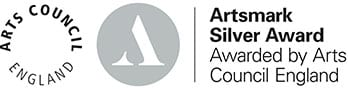# Further Mathematics

## Further Mathematics

Course content, structure & method of assessment

Further Mathematics is designed for students with an enthusiasm for mathematics, many of whom will go on to degrees in mathematics, engineering, the sciences and economics.

The qualification is deeper and broader than A level Mathematics.  A level Further Mathematics builds from GCSE and A level Mathematics. As well as building on algebra and calculus introduced in A level Mathematics, the core content introduces complex numbers and matrices, fundamental ideas with wide applications in engineering, physical sciences and computing. The non-core contents includes different options that can enable students to specialise in areas of mathematics that are particularly relevant to their interests and future aspirations. A level Further Mathematics prepares students for further study and employment in highly mathematical disciplines that require knowledge and understanding of sophisticated mathematical ideas and techniques.

### Why study A level Further Mathematics?

If you wish to follow:

• An engineering course
• A Maths related degree
• Any Medical course
• Any Science related course
• Accountancy/Economics

### Method of assessment

Year 12 Further Mathematics: Assessment is by 2 exams of 1 hour 30 mins.

• Paper 1 (80 marks) Content; Pure Mathematics: complex numbers, matrices, further algebra, further calculus, further vectors, polar coordinates and hyperbolic functions.
• Paper 2 (80 marks, two sections of 40 marks): Statistics and Mechanics: Statistics content; discrete random variables, Poisson distribution, type I and type II errors and power of a test and continuous random variables. Mechanics content; dimensional analysis, momentum and collisions, work power and energy and circular motion.

Year 13 Further Mathematics: Assessment is by 3 exams of 2 hours in duration.

• Paper 1: (100 marks): Content; Pure mathematics: proof, complex numbers, matrices, further algebra, further calculus, further vectors, polar coordinates, hyperbolic functions, differential equations, trigonometry, numerical methods and coordinate geometry are examinable.
• Paper 2: as above for paper 1
• Paper 3: (100 marks; two sections 50 marks each): Statistics and Mechanics: Statistics content; discrete random variables, Poisson distribution, type I and type II errors and power of a test, continuous random variables, exponential distribution and inference. Mechanics content; dimensional analysis, momentum and collisions, work power and energy and circular motion.

Level: A level

Board: AQA